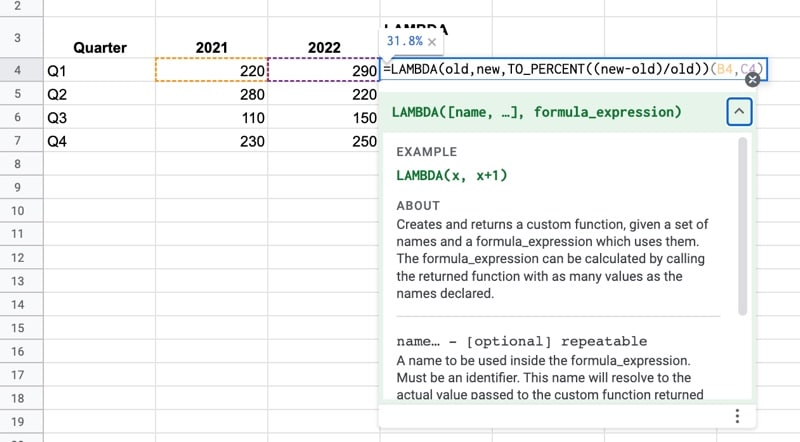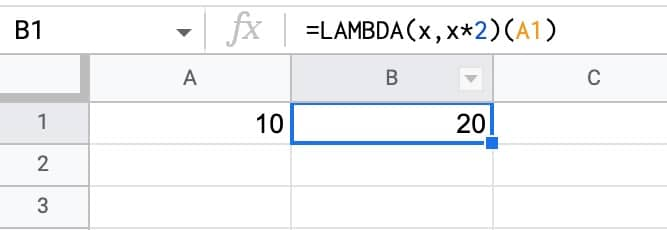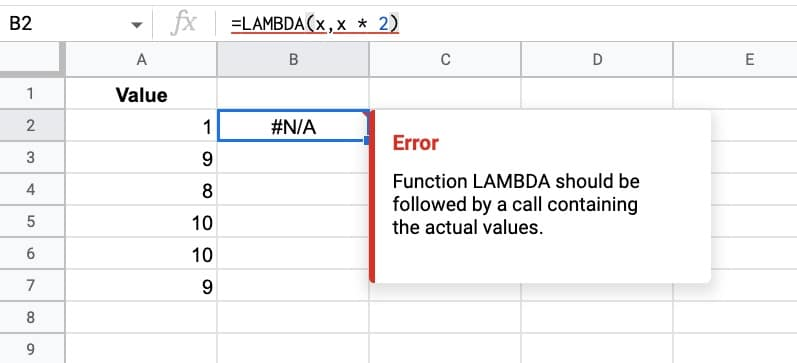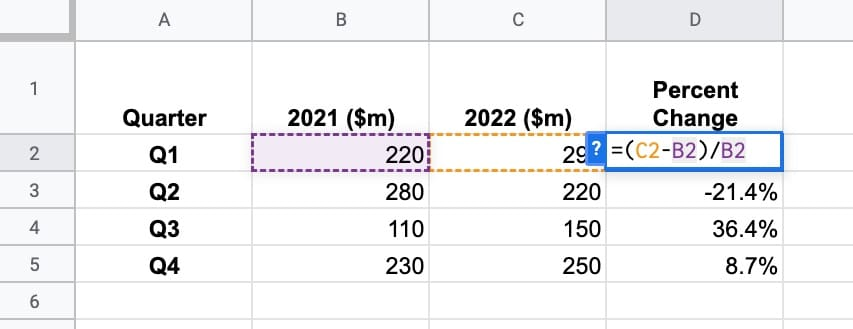# LAMBDA Function in Google Sheets

The LAMBDA function in Google Sheets creates a custom function with placeholder inputs, that can be reused.

The use case for the LAMBDA function is to work with other lambda helper functions, like MAP, REDUCE, SCAN, MAKEARRAY, BYROW, and BYCOL.

LAMBDA functions are also the underlying technology for Named Functions, which are hugely useful.

LAMBDA functions themselves are challenging to use and not very intuitive compared to regular functions.🔗 Get this example and others in the template at the bottom of this article.

Note: it may take up to 15 days from August 24th before you see lambda in your Sheets.

## Simple LAMBDA Function Example

We’ll start with the simplest LAMBDA function we can: a custom function to double a number.

I.e. x => 2x

The LAMBDA formula to double a number is:

`=LAMBDA(x,x*2)(A1)`

It works like so:Of course, it’s much easier to simply type `=A1 * 2` so this lambda is just to illustrate how it works.

## LAMBDA Function in Google Sheets: Syntax

The LAMBDA function takes one or more arguments:

`=LAMBDA([name,...], formula_expression)`

`[name,...]`

This is optional and repeatable and represents the placeholders to use inside your formula.

`formula_expression`

The formula definition to calculate. It can reference any placeholder names that have been defined.

Notes on using the LAMBDA Function

The LAMBDA function is the only function that takes two sets of brackets for inputs, i.e. it takes the form

`=LAMBDA(...)(...)`

where the first set of brackets define LAMBDA and the second set are the actual input values you want to use.

If you try to use LAMBDA without adding actual values or cell references after the definition, you’ll see the following error:## LAMBDA Percent Change Example

Suppose we have a table of quarterly financial data.

We want to calculate the percent change between 2021 and 2022, using this formula:

`=(C2-B2)/B2`

Which gives the following output:We can convert this to a LAMBDA formula by first defining the placeholders, then the function expression, and then supplying the actual values:

`=LAMBDA(oldVal,newVal,(newVal-oldVal)/oldVal)(B2,C2)`

Has that helped us?

No, not really!

The LAMBDA formula is more complex than the original formula.

But as I said at the beginning of this article, the LAMBDA function is really designed to work with the new lambda helper functions.

And they also underpin Named Functions, so they’re critical in that regard.

So, instead of a LAMBDA function, we could create a named function PERCENTCHANGE and use it as follows:

`=PERCENTCHANGE(B2,C2)`

Now that is easy!

## LAMBDA Helper Functions

As I mentioned earlier in this post, the main use case for the LAMBDA function is to work with the lambda helper functions:

• MAP: Returns a new array formed by mapping each value in array_or_range to a new value
• REDUCE: Reduces an array_or_range by repeatedly applying a lambda to each value in array_or_range
• BYCOL: Returns a new row array formed by grouping each column in array_or_range to a single value
• BYROW: Returns a new column array formed by grouping each row in array_or_range to a single value
• SCAN: Scans array_or_range by repeatedly applying a lambda to each value in array_or_range
• MAKEARRAY: Returns a calculated array of specified rows and columns

## LAMBDA Template

Feel free to make a copy: File > Make a copy…

If you can’t access the template, it might be because of your organization’s Google Workspace settings.

In this case, right-click the link to open it in an Incognito window to view it.

## 10 thoughts on “LAMBDA Function in Google Sheets”

1.Mike says:

So I seem to be getting these new functions slowly. I have XLOOKUP and LAMBDA on some sheet but not Named Functions. So without named functions there’s really no point to LAMBDA, right? Or am I missing something.

1.Ben says:

Sort of…

You wouldn’t use a lambda on its own because named functions are way easier, but lambda is super useful when combined with the helper functions like MAP, REDUCE, etc.

2.MikeJ says:

This thread may be too old for anybody to read, but I discovered something interesting about how rand() works when used in a Lambda function. The same is probably true of today(), now() and other functions that recalculate every time any cell is changed.

First, any random number generated with Lambda can be used multiple times in a formula. That is not true with when using rand() outside of Lambda. For example, consider the following two formulas:
=lambda(r,if(r<0.5,{"small",r},{"large",r}))(rand())
=if(rand()<0.5,{"small",rand()},{"large",rand()})
The goal is to print "small" or "large," as appropriate, followed by the random number. The second formula will not work as intended, because it generates two random numbers, and the second random number may not match the "small" or "large" designation. Lambda lets you save the random number, like a variable, and use it more than once in a single cell formula, which allows the first formula above to work as intended.

You might want a formula like this (more complicate, obviously) to generate a skewed random distribution, such as a triangular distribution for a Monte Carlo simulation.

Second, the rand() function when inside Lambda does not recalculate every time a cell is change. In fact, it appears not to recalculate even when you refresh the browser. If today() and now() work the same, then it won't be hard to think of some possible simple uses for Lambda.

3.Daniele says:

Today is September 6, 2022 and I am unable to get any of these functions to work. The examples from your file also don’t work. Am I having problems or are those new features still not working?

1.MikeJ says:

It’s not just you. Google appears to have retracted access to all of the new functions that were previously released, at least that’s the case for both my personal Google account and my Workspace account. They must have found a bug.

1.Ben says:

Thanks for the heads up, Mike! I saw this too and had it confirmed. They’ll be back soon hopefully. Interestingly I still have access to them ‍♂️

4.Daniele says:

to be more precise, the error is: invalid call to a non-function

5.Daniele says:

Today is September 7, 2022 and finally the lambda function works 🙂

1.Ben says:

Woohoo!

6.Sunil kumar says:

very nice